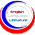Get your Assignment, Term Paper and Project Work on English and Literature Done by Contacting this Blog...

# Easy Way to Calculate JAMB/Post UTME Admission Screening Scores

Did you know that:

1. Candidates who score above 250 will gain merit admission by JAMB into their preferred institutions?

2.  The duration of the 2017 JAMB CBT is three (3) years for candidates with a score of 200 and above?

3. Scholarship may be awarded to candidates with a score of 280 and above in various institutions for a 4-year degree program.

Having known that, let's go to the crux of the matter.

Last year, The Joint Admissions and Matriculation Board, JAMB, approved a new JAMB/Post-UTME admission screening system for all candidates seeking admission into various tertiary institutions in the country. It was called JAMB Point-System.

That was necessary following the scrapping of Post-UTME test by the Ministry of Education. It is now expected that the new admission screening system will take full effect during the 2017/2018 academic session admission exercise.
In order to keep candidates on the right track, I decided to lecture them on how to calculate Post-UTME admission screening scores with their O’level resuts, either WAEC or NECO.

Below is a breakdown of JAMB's Post UTME screening system.

POST UTME SCREENING SYSTEM

1. O'Level Result: 40%

JAMB apportions 40% for O'level result. A candidate who made five As in WAEC or NECO, automatically gets 40%.

See more breakdown below:
• A1 = 8%
• B2 = 7%
• B3 = 6%
• C4 = 5%
• C5 = 4%
• C6 = 3%
Therefore:
• Five A1 = 8% x 5 = 40%
• Five B2 = 7% x 5 = 35%
• Five B3 = 6% x 5 = 30%
• Five C4 = 5% x 5 = 25%
• Five C5 = 4% x 5 = 20%
• Five C6 = 3% x 5 = 15%
2. UTME SCORES = 60%

JAMB apportions 60% for UTME and only candidates who scored 230 and above are qualified for this mark. Take a look at the breakdown.
• 180-189 = 10%
• 190-199 = 20%
• 200-209 = 30%
• 210-219 = 40%
• 220-229 = 50%
• 230 and above = 60%
Let's try a working example

Tammy wrote WAEC or NECO and had the following grades in these relevant five subjects:
• Mathematics = B2
• English = A1
• Literature = C4
• Government = B3
• C.R.S = C5
Tammy's score will therefore be:
• Mathematics = 7%
• English = 8%
• Literature = 5%
• Government  = 6%
• C.R.S = 4%
Making a total of 30%

Tammy then scored 220 in his JAMB examination and from the above explanation, 220 falls under 50%.

Therefore, Tammy's Post UTME score = His total WAEC/NECO Percentage + His JAMB percentage.

That is: 30% + 50% = 80%.

Tammy's Post UTME score is now 80%. Therefore, Tammy's chance of gaining admission this year is very high.

#### 2 comments:

1.Anonymous01 July, 2017

I sumed mine chance of gaining admission which 60%. Is it enough for me to be admitted into the university.

1.